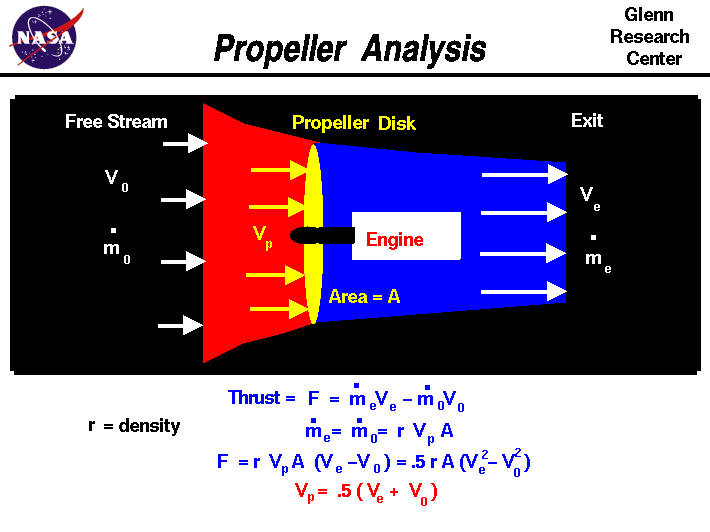+ Text Only Site
+ Non-Flash Version
+ Contact GlennMost general aviation or private airplanes are powered by internal combustion engines which turn propellers to generate thrust. The details of how a propeller generates thrust is very complex, but we can still learn a few of the fundamentals using the simplified momentum theory presented here. Propeller Propulsion System On the slide, we show a schematic of a propeller propulsion system at the top and some of the equations that define how a propeller produces thrust at the bottom. The details of propeller propulsion are very complex because the propeller is like a rotating wing. Propellers usually have between 2 and 6 blades. The blades are usually long and thin, and a cut through the blade perpendicular to the long dimension will give an airfoil shape. Because the blades rotate, the tip moves faster than the hub. So to make the propeller efficient, the blades are usually twisted. The angle of attack of the airfoils at the tip is lower than at the hub because it is moving at a higher velocity than the hub. Of course, these variations make analyzing the airflow through the propeller a verydifficult task. Leaving the details to the aerodynamicists, let us assume that the spinning propeller acts like a disk through which the surrounding air passes (the yellow ellipse in the schematic). The engine, shown in white, turns the propeller and does work on the airflow. So there is an abrupt change in pressure across the propeller disk. The propeller acts like a rotating wing. From airfoil theory, we know that the pressure over the top of a lifting wing is lower than the pressure below the wing. A spinning propeller sets up a pressure lower than free stream in front of the propeller and higher than free stream behind the propeller. Downstream of the disk the pressure eventually returns to free stream conditions. But at the exit, the velocity is greater than free stream because the propeller does work on the airflow. We can apply Bernoulli'sequation to the air in front of the propeller and to the air behind the propeller. But we cannot apply Bernoulli's equation across the propeller disk because the work performed by the engine violates an assumption used to derive the equation. Simple Momentum Theory Turning to the math, from the basic thrust equation, we know that the amount of thrust depends on the mass flow rate through the propeller and the velocity change through the propulsion system. Let us denote the free stream conditions by the subscript "0", the conditions at the propeller by the subscript "p", and the exit conditions by the subscript "e". The thrust F is equal to the mass flow rate m dot times the difference in velocity V. F = [m dot * V]e - [m dot * V]0 There is no pressure-area term because the pressure at the exit is equal to the free stream pressure. The mass flow through the propulsion system is a constant, and we can determine the value at the plane of the propeller. Since the propeller rotates, we can define an area A that is swept out by the propeller of blade length L. Through this area, the mass flow rate is density r times velocity Vp, times area. m dot = r * Vp * A Substitute this value for the mass flow rate into the thrust equation to get thethrust in terms of the exit velocity, entrance velocity, and velocity through the propeller. F = r * Vp * A * [Ve - V0] On another page, we have determined that the thrust is related to the pressure jump across the propeller disk. Using Bernoulli's equation upstream and downstream of the propeller, we obtain: F = .5 * r * A * [Ve ^2 - V0 ^2] Combining the two expressions for the the thrust F and solving for Vp; Vp = .5 [Ve + V0] The airspeed through the propeller disk is simply the average of the free stream and exit velocities. Note that this thrust is an ideal number that does not account for many losses that occur in practical, high speed propellers like tip losses. The losses must be determined by a more detailed propeller theory, which is beyond the scope of these pages. The complex theory also provides the magnitude of the pressure jump for a given geometry. The simple momentum theory, however, provides a good first cut at the answer and could be used for a preliminary design. Activities: Guided Tours Navigation ..Beginner's Guide Home Page+ Inspector General Hotline + Equal Employment Opportunity Data Posted Pursuant to the No Fear Act + Budgets, Strategic Plans and Accountability Reports + Freedom of Information Act + The President's Management Agenda + NASA Privacy Statement, Disclaimer, and Accessibility CertificationEditor: Nancy Hall NASA Official: Nancy Hall Last Updated: May 13 2021 + Contact Glenn